Equations > Trigonometry > Trigonometric Identities

Showing results 1 to 10 of 11, on page 1 of 2
Go to page: 1 2
 Description Equation Double Angle Identity - Sine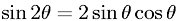Double Angle Identity - Cosine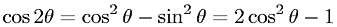Half Angle Identity - Sine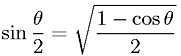Half Angle Identity - Cosine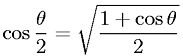Sum and Difference of Angles Identity - Sine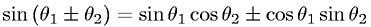Sum and Difference of Angles Identity - Cosine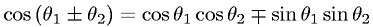Sum and Difference of Angles Identity - TangentSuperposition (Addition and Subtraction) of Sine of Angles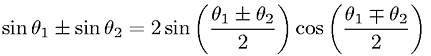Superposition (Addition) of Cosine of Angles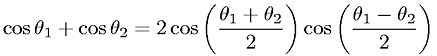Superposition (Subtraction) of Cosine of Angles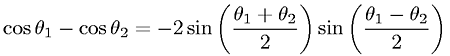(you need to login first) Go to page: 1 2